# NCERT Solutions - Probability JEE Notes | EduRev

## JEE : NCERT Solutions - Probability JEE Notes | EduRev

The document NCERT Solutions - Probability JEE Notes | EduRev is a part of the JEE Course Mathematics (Maths) Class 12.
All you need of JEE at this link: JEE

Exercise 13.1

Question 1:

Given that E and F are events such that P(E) = 0.6, P(F) = 0.3 and P(E ∩ F) = 0.2, find P (E|F) and P(F|E).

It is given that P(E) = 0.6, P(F) = 0.3, and P(E ∩ F) = 0.2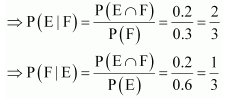Question 2:

Compute P(A|B), if P(B) = 0.5 and P (A ∩ B) = 0.32

It is given that P(B) = 0.5 and P(A ∩ B) = 0.32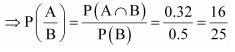Question 3:

If P(A) = 0.8, P(B) = 0.5 and P(B|A) = 0.4, find

(i) P(A ∩ B) (ii) P(A|B) (iii) P(A ∪ B)

It is given that P(A) = 0.8, P(B) = 0.5, and P(B|A) = 0.4

(i) P (B|A) = 0.4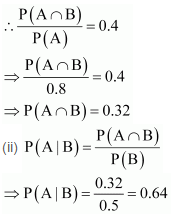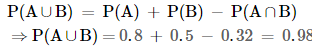Question 4:

Evaluate P (A ∪ B), if 2P (A) = P (B) = 5/13 and P(A|B) = 2/5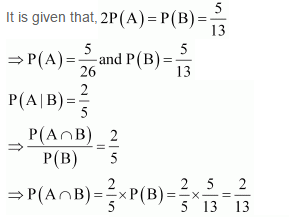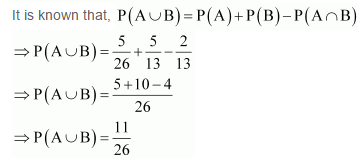Question 5:

If P(A) = 6/11, P(B) =5/11 and P(A ∪ B) =7/11 , find

(i) P(A ∩ B) (ii) P(A|B) (iii) P(B|A)

It is given that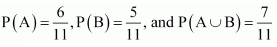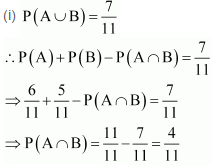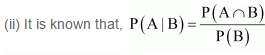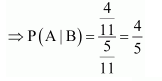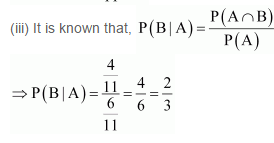Question 6:

A coin is tossed three times, where

(i) E: head on third toss, F: heads on first two tosses

(iii) E: at most two tails, F: at least one tail

If a coin is tossed three times, then the sample space S is

S = {HHH, HHT, HTH, HTT, THH, THT, TTH, TTT}

It can be seen that the sample space has 8 elements.

(i) E = {HHH, HTH, THH, TTH}

F = {HHH, HHT}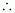E ∩ F = {HHH}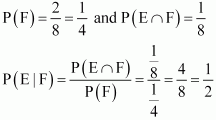(ii) E = {HHH, HHT, HTH, THH}

F = {HHT, HTH, HTT, THH, THT, TTH, TTT}E ∩ F = {HHT, HTH, THH}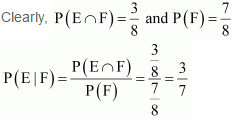(iii) E = {HHH, HHT, HTT, HTH, THH, THT, TTH}

F = {HHT, HTT, HTH, THH, THT, TTH, TTT}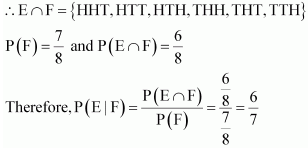Question 7:

Two coins are tossed once, where

(i) E: tail appears on one coin, F: one coin shows head

(ii) E: not tail appears, F: no head appears

If two coins are tossed once, then the sample space S is

S = {HH, HT, TH, TT}

(i) E = {HT, TH}

F = {HT, TH}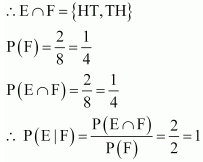(ii) E = {HH}

F = {TT}

∴ E ∩ F = Φ

P (F) = 1 and P (E ∩ F) = 0

∴ P(E|F) =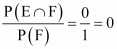Question 8:

A die is thrown three times,

E: 4 appears on the third toss, F: 6 and 5 appears respectively on first two tosses

If a die is thrown three times, then the number of elements in the sample space will be 6 × 6 × 6 = 216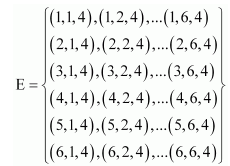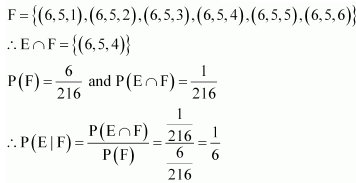Question 9:

Mother, father and son line up at random for a family picture

E: son on one end, F: father in middle

If mother (M), father (F), and son (S) line up for the family picture, then the sample space will be

S = {MFS, MSF, FMS, FSM, SMF, SFM}

⇒ E = {MFS, FMS, SMF, SFM}

F = {MFS, SFM}

∴ E ∩ F = {MFS, SFM}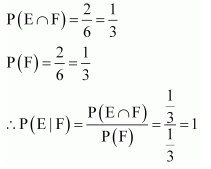Question 10:

A black and a red dice are rolled.

(a) Find the conditional probability of obtaining a sum greater than 9, given that the black die resulted in a 5.

(b) Find the conditional probability of obtaining the sum 8, given that the red die resulted in a number less than 4.

Let the first observation be from the black die and second from the red die.

When two dice (one black and another red) are rolled, the sample space S has 6 × 6 = 36 number of elements.
Let A: Obtaining a sum greater than 9

= {(4, 6), (5, 5), (5, 6), (6, 4), (6, 5), (6, 6)}

B: Black die results in a 5.

= {(5, 1), (5, 2), (5, 3), (5, 4), (5, 5), (5, 6)}

∴ A ∩ B = {(5, 5), (5, 6)}

The conditional probability of obtaining a sum greater than 9, given that the black die resulted in a 5, is given by P (A|B).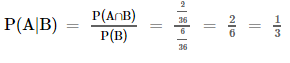(b) E: Sum of the observations is 8.

= {(2, 6), (3, 5), (4, 4), (5, 3), (6, 2)}

F: Red die resulted in a number less than 4.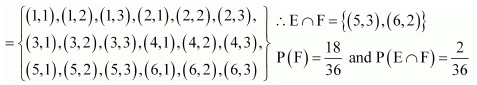The conditional probability of obtaining the sum equal to 8, given that the red die resulted in a number less than 4, is given by P (E|F).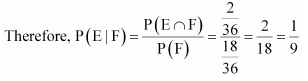Question 11:

A fair die is rolled. Consider events E = {1, 3, 5}, F = {2, 3} and G = {2, 3, 4, 5}

Find (i) P (E|F) and P (F|E) (ii) P (E|G) and P (G|E) (ii) P ((E ∪ F)|G) and P ((E ∩ G)|G)

When a fair die is rolled, the sample space S will be

S = {1, 2, 3, 4, 5, 6}

It is given that E = {1, 3, 5}, F = {2, 3}, and G = {2, 3, 4, 5}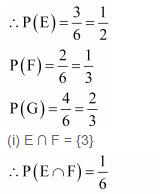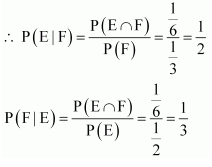(ii) E ∩ G = {3, 5}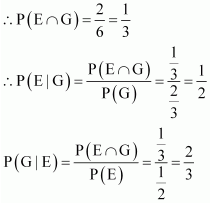(iii) E ∪ F = {1, 2, 3, 5}

(E ∪ F) ∩ G = {1, 2, 3, 5} ∩{2, 3, 4, 5} = {2, 3, 5}

E ∩ F = {3}

(E ∩ F) ∩ G = {3}∩{2, 3, 4, 5} = {3}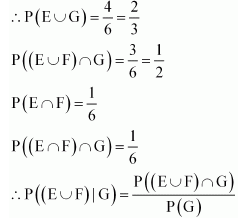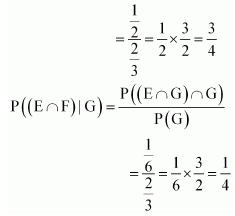Question 12:

Assume that each born child is equally likely to be a boy or a girl. If a family has two children, what is the conditional probability that both are girls given that (i) the youngest is a girl, (ii) at least one is a girl?

Let b and g represent the boy and the girl child respectively. If a family has two children, the sample space will be

S = {(bb), (bg), (gb), (g, g)}

Let A be the event that both children are girls.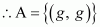(i) Let B be the event that the youngest child is a girl.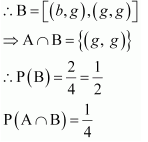The conditional probability that both are girls, given that the youngest child is a girl, is given by P (A|B).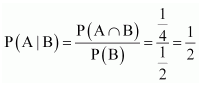Therefore, the required probability is (1/2).

(ii) Let C be the event that at least one child is a girl.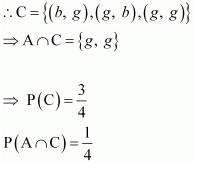The conditional probability that both are girls, given that at least one child is a girl, is given by P(A|C).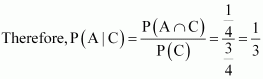Question 13:

An instructor has a question bank consisting of 300 easy True/False questions, 200 difficult True/False questions, 500 easy multiple choice questions and 400 difficult multiple choice questions. If a question is selected at random from the question bank, what is the probability that it will be an easy question given that it is a multiple choice question?

The given data can be tabulated as

 True/False Multiple choice Total Easy 300 500 800 Difficult 200 400 600 Total 500 900 1400

Let us denote E = easy questions, M = multiple choice questions, D = difficult questions, and T = True/False questions

Total number of questions = 1400

Total number of multiple choice questions = 900

Therefore, probability of selecting an easy multiple choice question is

P (E ∩ M) = 500/1400= 5/14

Probability of selecting a multiple choice question, P (M), is 900/1400= 9/14

P (E|M) represents the probability that a randomly selected question will be an easy question, given that it is a multiple choice question.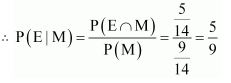Therefore, the required probability is 5/9

Question 14:

Given that the two numbers appearing on throwing the two dice are different. Find the probability of the event ‘the sum of numbers on the dice is 4’.

When dice is thrown, number of observations in the sample space = 6 × 6 = 36

Let A be the event that the sum of the numbers on the dice is 4 and B be the event that the two numbers appearing on throwing the two dice are different.
∴ A = {(1, 3), (2, 2), (3, 1)}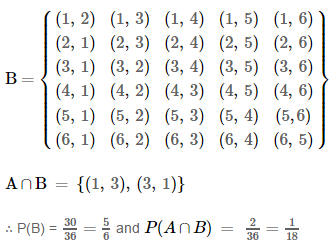Let P (A|B) represent the probability that the sum of the numbers on the dice is 4, given that the two numbers appearing on throwing the two dice are different.

Question 15:

Consider the experiment of throwing a die, if a multiple of 3 comes up, throw the die again and if any other number comes, toss a coin. Find the conditional probability of the event ‘the coin shows a tail’, given that ‘at least one die shows a 3’.

The outcomes of the given experiment can be represented by the following tree diagram.

The sample space of the experiment is,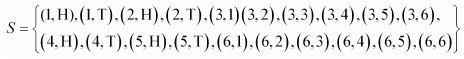Let A be the event that the coin shows a tail and B be the event that at least one die shows 3.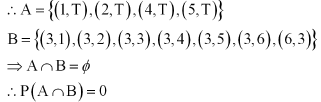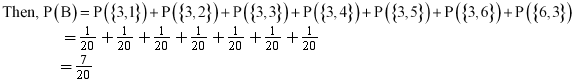Probability of the event that the coin shows a tail, given that at least one die shows 3, is given by P(A|B).

Therefore,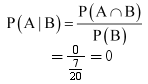Question 16:

If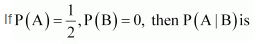(A) 0 (B) (1/2)

(C) not defined (D) 1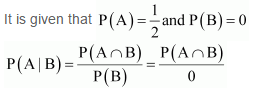Therefore, P (A|B) is not defined.

Thus, the correct answer is C.

Question 17:

If A and B are events such that P (A|B) = P(B|A), then

(A) A ⊂ B but A ≠ B (B) A = B

(C) A ∩ B = Φ (D) P(A) = P(B)

It is given that, P(A|B) = P(B|A)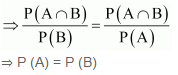Thus, the correct answer is D.

### Exercise 13.2

Question 1:find P (A ∩ B) if A and B are independent events.

It is given that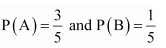A and B are independent events. Therefore,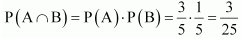Question 2:

Two cards are drawn at random and without replacement from a pack of 52 playing cards. Find the probability that both the cards are black.

There are 26 black cards in a deck of 52 cards.

Let P (A) be the probability of getting a black card in the first draw.

P(A) = 26/52 = 1/2

Let P (B) be the probability of getting a black card on the second draw.

Since the card is not replaced,

P(B) =25/51

Thus, probability of getting both the cards black =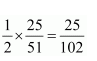Question 3:

A box of oranges is inspected by examining three randomly selected oranges drawn without replacement. If all the three oranges are good, the box is approved for sale, otherwise, it is rejected. Find the probability that a box containing 15 oranges out of which 12 are good and 3 are bad ones will be approved for sale.

Let A, B, and C be the respective events that the first, second, and third drawn orange is good.

Therefore, probability that first drawn orange is good, P(A) = 12/15

The oranges are not replaced.

Therefore, probability of getting second orange good, P(B) = 11/14

Similarly, probability of getting third orange good, P(C) = 10/3

The box is approved for sale, if all the three oranges are good.

Thus, probability of getting all the oranges good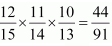Therefore, the probability that the box is approved for sale is 44/91.

Question 4:

A fair coin and an unbiased die are tossed. Let A be the event ‘head appears on the coin’ and B be the event ‘3 on the die’. Check whether A and B are independent events or not.

If a fair coin and an unbiased die are tossed, then the sample space S is given by,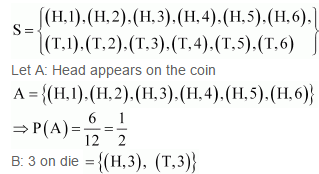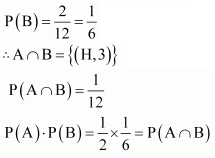Therefore, A and B are independent events.

Question 5:

A die marked 1, 2, 3 in red and 4, 5, 6 in green is tossed. Let A be the event, ‘the number is even,’ and B be the event, ‘the number is red’. Are A and B independent?

When a die is thrown, the sample space (S) is

S = {1, 2, 3, 4, 5, 6}

Let A: the number is even = {2, 4, 6}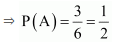B: the number is red = {1, 2, 3}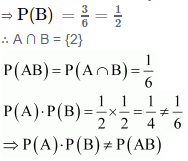Therefore, A and B are not independent.

Question 6:

Let E and F be events with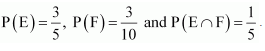Are E and F independent?

Answer 6: It is given that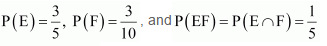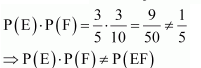Therefore, E and F are not independent.

Question 7:

Given that the events A and B are such that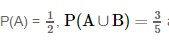and P (B) = p. Find p if they are (i) mutually exclusive (ii) independent.

It is given that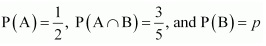(i) When A and B are mutually exclusive, A ∩ B = Φ

∴ P (A ∩ B) = 0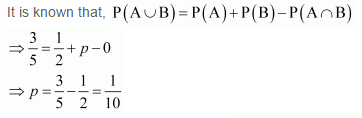(ii) When A and B are independent,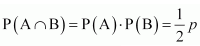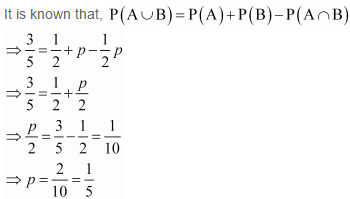Question 8:

Let A and B be independent events with P (A) = 0.3 and P (B) = 0.4. Find

(i) P (A ∩ B) (ii) P (A ∪ B)

(iii) P (A|B) (iv) P (B|A)

It is given that P (A) = 0.3 and P (B) = 0.4

(i) If A and B are independent events, then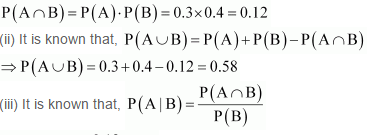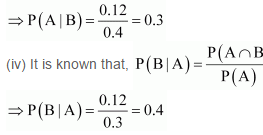Question 9:

If A and B are two events such that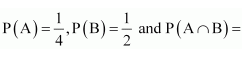find P (not A and not B).

It is given that,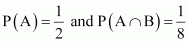P(not on A and not on B) =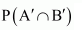P(not on A and not on B) =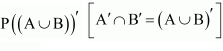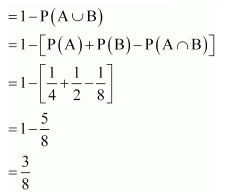Question 10:

Events A and B are such that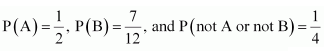State whether A and B are independent?

It is given that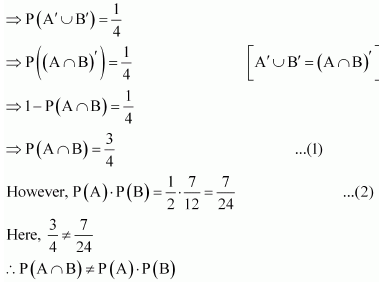Therefore, A and B are not independent events.

Question 11:

Given two independent events A and B such that P (A) = 0.3, P (B) = 0.6. Find

(i) P (A and B) (ii) P (A and not B)

(iii) P (A or B) (iv) P (neither A nor B)

It is given that P (A) = 0.3 and P (B) = 0.6

Also, A and B are independent events.

Also, A and B are independent events.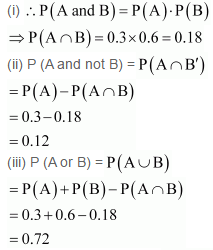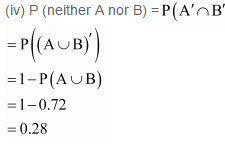Question 12:

A die is tossed thrice. Find the probability of getting an odd number at least once.

Probability of getting an odd number in a single throw of a die 3/6= 1/2

Similarly, probability of getting an even number = 3/6 = 1/2

Probability of getting an even number three times =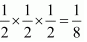Therefore, probability of getting an odd number at least once

= 1 − Probability of getting an odd number in none of the throws

= 1 − Probability of getting an even number thrice

=1-1/8= 7/8

Offer running on EduRev: Apply code STAYHOME200 to get INR 200 off on our premium plan EduRev Infinity!

## Mathematics (Maths) Class 12

209 videos|222 docs|124 tests

,

,

,

,

,

,

,

,

,

,

,

,

,

,

,

,

,

,

,

,

,

;